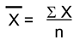# numpy.mean() vs numpy.average() in NumPy

Learn about the difference between numpy.mean() and numpy.average() methods?
Submitted by Pranit Sharma, on December 21, 2022

NumPy is an abbreviated form of Numerical Python. It is used for different types of scientific operations in python. Numpy is a vast library in python which is used for almost every kind of scientific or mathematical operation. It is itself an array which is a collection of various methods and functions for processing the arrays.

## numpy.mean() vs numpy.average()

Although both of these methods result in the same value, we need to understand the difference between the working of these methods.

Both numpy.mean() and numpy.average() methods are used to find the mean value of a NumPy array.

Mean is nothing but an average value of a series of a number. Mathematically, the mean can be calculated as:Here, is the mean, ∑x is the summation of all the values and n is the total number of values/elements.

Suppose we have a series of numbers from 1 to 10, then the average of this series will be:

```∑x = 1+2+3+4+5+6+7+8+9+10
∑x = 55
n = 10
x̄ = 55/10
x̄ = 5.5
```

Hence, by using numpy.mean() or numpy.average() for a NumPy array, e.g. [1,2,3,4,5,6,7,8,9,10], the result would be 55.

• The numpy.mean() always computes an arithmetic mean, and has some additional options for input and output (e.g., what datatypes to use, where to place the result).
• The numpy.average() can compute a weighted average if the weights parameter is supplied.
• The numpy.mean() takes into account masks, so compute the mean only over unmasked values.
• The numpy.average() does not take into account masks, so compute the average over the whole set of data.

In addition to these differences, another extremely important difference is that numpy.average() does not allow the dtype keyword, which is essential for getting correct results in some cases while numpy.mean() does.